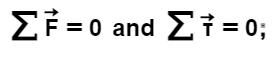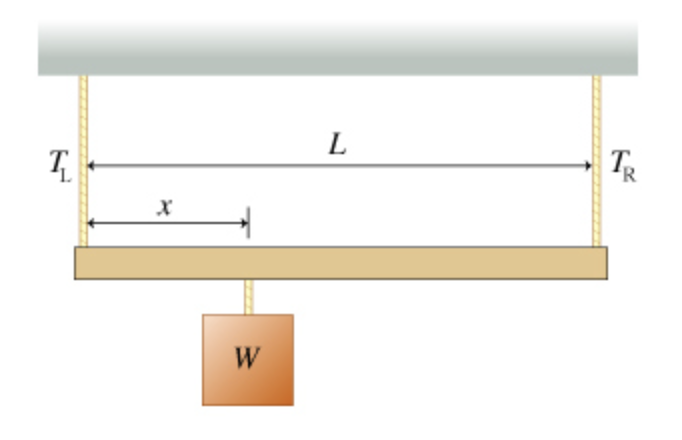# Problem: To understand the conditions necessary for static equilibrium. Look around you, and you see a world at rest. The monitor, desk, and chair–and the building that contains them–are in a state described as static equilibrium. Indeed, it is the fundamental objective of many branches of engineering to maintain this state in spite of the presence of obvious forces imposed by gravity and static loads or the more unpredictable forces from wind and earthquakes. The condition of static equilibrium is equivalent to the statement that the bodies involved have neither linear nor angular acceleration. Hence static mechanical equilibrium (as opposed to thermal or electrical equilibrium) requires that the forces acting on a body simultaneously satisfy two conditions: A. Solve for TR, the tension in the right rope. (Express your answer in terms of W and the dimensions L and x. Not all of these variables may show up in the solution.)B. Solve for TL, the tension in the left rope. (Express your answer in terms of W and the dimensions L and x. Not all of these variables may show up in the solution.)

###### FREE Expert Solution

At the left end, $\overline{){\mathbf{\sum }}{\mathbf{\tau }}{\mathbf{=}}{\mathbf{0}}}$

A.

The total torque, ΣΤ:

83% (176 ratings)###### Problem Details

To understand the conditions necessary for static equilibrium.

Look around you, and you see a world at rest. The monitor, desk, and chair–and the building that contains them–are in a state described as static equilibrium. Indeed, it is the fundamental objective of many branches of engineering to maintain this state in spite of the presence of obvious forces imposed by gravity and static loads or the more unpredictable forces from wind and earthquakes.

The condition of static equilibrium is equivalent to the statement that the bodies involved have neither linear nor angular acceleration. Hence static mechanical equilibrium (as opposed to thermal or electrical equilibrium) requires that the forces acting on a body simultaneously satisfy two conditions:A. Solve for TR, the tension in the right rope. (Express your answer in terms of W and the dimensions L and x. Not all of these variables may show up in the solution.)

B. Solve for TL, the tension in the left rope. (Express your answer in terms of W and the dimensions L and x. Not all of these variables may show up in the solution.)